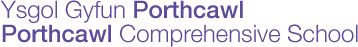Generic selectors
Exact matches only
Search in title
Search in content
Post Type Selectors

# Maths

Miss E Pugh (Head of Department and Head of the Faculty of Maths, ICT & Electronics)

• Key Stage 3

### Year 7

#### Term 1

Fractions, Decimals, Percentages
Angles 0º to 360º; acute, obtuse, reflex
Area and perimeter
Rectangles, triangles and parallelograms
Includes compound shapes
Angles on a straight line; at a point; vertically opposite
Angles of a triangle; equilateral/isosceles
Mean, median, mode of discrete data
Interpret graphs/diagrams; pictograms; pie charts

#### Term 2

Algebra Notation, HCF and LCM – Factors and prime numbers, Prime factors
Ratio
Venn diagrams
Simple algebraic equations
Scale 0-1, words of probability
Fractions +/-

#### Term 3

Nth term of a sequence

Numeracy topics are studied throughout the year by multi-stage problem solving. Questions are:
– Division
– Long multiplication
– Negative numbers
– Probability
– Quality of written communication
– Ratio
– Time

During the course of the year we work towards improving standards in procedural and reasoning Government tests by way of starters and concentrating on problem solving questions.

### Year 8

#### Term 1 Intermediate – Syllabus A (Set 1/2/3)

Multiplying and dividing decimals
BODMAS
Using a calculator
Percentages and ratio
Perimeter, area, volume and density
Interior and exterior angles of a polygon
Co-ordinates and plotting graphs

#### Term 1 Foundation – Syllabus B (Set 4/5)

Multiplying and dividing by 10, 100, 1000 etc
Long multiplication and division (Numeracy)
Simplifying algebraic expressions, expanding single brackets, substitution and simple equations/inequalities
Probability
Pie charts/scatter graphs/line graphs/revise pie charts
Negative numbers

#### Term 2 Intermediate – Syllabus A (Set 1/2/3)

Number patterns and finding the nth term
Equations
Grouped frequency diagrams, frequency polygons, estimated mean and scatter graphs
Fractions
Probability trees
Solving inequalities

#### Term 2 Foundation – Syllabus B (Set 4/5)

Perimeter, area and volume
Enlarge with whole number scale factor
Reflective and rotational symmetry
Angles on parallel lines
Polygons
Tessellations/congruent shapes
Converting between decimals, fractions and percentages
Understand compound interest
Percentage increase, decrease/use ratio
Co-ordinates
Bearings

#### Term 3 Intermediate – Syllabus A (Set 1/2/3)

Unit conversion
Multiplying, dividing and zero indices
Angles to parallel lines
Travel graphs

#### Term 3 Foundation – Syllabus B (Set 4/5)

Grouped data
Mean/median/mode/range grouped data
Sequences

Numeracy topics are studied throughout the year by multi-stage problem solving. Questions are:
– Money
– Fractions/decimals/percentages
– Using a calculator
– Shape and space
– Probability
– Time and time zones
– Quality of written communication

During the course of the year we work towards improving standards in procedural and reasoning Government tests by way of starters and concentrating on problem solving questions.

### Year 9

#### Term 1 Higher (Set 1/2)

Cumulative frequency
Box and whisker graphs
Stratified sampling
Standard form
Product of prime factors
Rounding, upper and lower bounds
Co-ordinates, graph work and solving equations from graphs
Pythagoras theorem

#### Term 1 Intermediate (Set 3/4)

Cumulative frequency
Box and whisker graphs
Rounding, upper and lower bounds
Estimation
Pythagoras theorem
Reverse percentage
Compound and simple interest

#### Term 2 Higher (Set 1/2)

Trigonometry – right angled only
Reverse percentage
Compound interest
Fractions
Simultaneous equations
Transformations

#### Term 2 Intermediate (Set 3/4)

Simultaneous equations
Transformations
Loci
Co-ordinates and graph work

#### Term 3 Higher (Set 1/2)

Similar triangles
Loci
Algebra – change the subject
Rational and irrational numbers
Indices and surds

#### Term 3 Intermediate (Set 3/4)

Standard form
Trigonometry

Numeracy topics are studied throughout the year by multi-stage problem solving. Questions are:
– Bearings and loci
– Formulae
– Money
– Ratio
– Shape and space
– Percentage and fractional change
– Comparing and analysing data from tables

During the course of the year we work towards improving standards in procedural and reasoning Government tests by way of starters and concentrating on problem solving questions.

#### Ways in which parents can help:

1. Year 7 pupils need to learn their times tables in the following fashion: 1×8=8, 2×8=16… up to the 12 times table.
2. Year 7 pupils must show their workings out to all questions.
3. Year 7 pupils should look in their book at worked examples to help them with class work and homework.
4. Practice times tables with pupils at home.
5. Practice basic numeracy and mental maths around the home and shopping e.g. money.
6. Encourage pupils to use the school resource centre at home.
7. Check pupils’ books regularly for progress comments and completion of work. Do the basics and check for good mental arithmetic (knowledge of tables) and when shopping etc working out best out purchases and discounts advertised in shops.
8. If the pupil has fallen behind or stuck on a topic to encourage pupil to attend extra help session on a Thursday lunchtime with nominated teacher; Alternatively arrange a time with classroom teacher.

#### Useful books/magazines/publications:

1. Any KS3 mathematic books/revision guides
2. Any logical puzzle books e.g. Sudoku
3. KS3 text books Exploring maths
4. There are good maths games on the market such as Big Brain Training

## Latest News

• ### School Show 2024

This year the school can reveal that they will be performing Jekyll & Hyde...

• ### UK Mathematics Trust Senior Maths Challenge – PCS Success!

Ten Math’s students from Porthcawl Comprehensive School achieved certificates in the UK’s Mathematics Trust...

• ### GCSE Results 2023

GCSE Excellence continues at Porthcawl Comprehensive School We are delighted with the outstanding results...